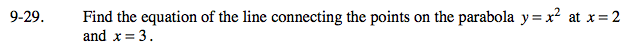### Home > PCT > Chapter Ch9 > Lesson 9.1.2 > Problem9-29

9-29.

Find the equation of the line connecting the points on the parabola y = x2 at x = 2 and x = 3. Homework Help ✎1. Determine the (x, y) coordinates of the points of interest.
2. Calculate the slope between the two points.
3. Using one of the points and the slope, write the equation.